# What is Exploratory Data Analysis ?

• Difficulty Level : Hard
• Last Updated : 22 Jul, 2021

Exploratory Data Analysis (EDA) is an approach to analyze the data using visual techniques. It is used to discover trends, patterns, or ti check assumptions with the help of statistical summary and graphical representations.

## Dataset Used

For the simplicity of the article, we will use a single dataset. We will use the employee data for this. It contains 8 columns namely – First Name, Gender, Start Date, Last Login, Salary, Bonus%, Senior Management, and Team.

Attention geek! Strengthen your foundations with the Python Programming Foundation Course and learn the basics.

To begin with, your interview preparations Enhance your Data Structures concepts with the Python DS Course. And to begin with your Machine Learning Journey, join the Machine Learning - Basic Level Course

Dataset Used: Employees.csv

Let’s read the dataset using the Pandas module and print the 1st five rows. To print the first five rows we will use the head() function.

Example:

## Python3

 `import` `pandas as pd``import` `numpy as np`` ` `df ``=` `pd.read_csv(``'employees.csv'``)``df.head()`

Output: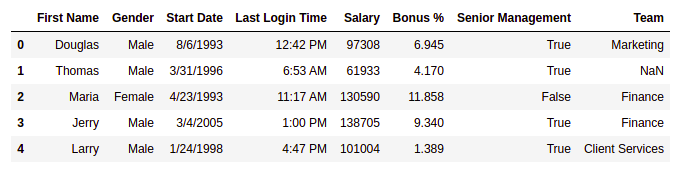## Getting insights about the dataset

Let’s see the shape of the data using the shape.

Example:

## Python3

 `df.shape`

Output:

`(1000, 8)`

This means that this dataset has 1000 rows and 8 columns.

Let’s get a quick summary of the dataset using the describe() method. The describe() function applies basic statistical computations on the dataset like extreme values, count of data points standard deviation, etc. Any missing value or NaN value is automatically skipped. describe() function gives a good picture of the distribution of data.

Example:

## Python3

 `df.describe()`

Output: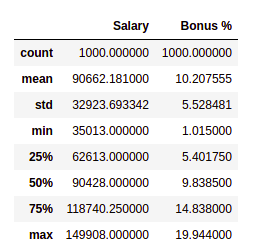Now, let’s also the columns and their data types. For this, we will use the info() method.

## Python3

 `df.info()`

Output: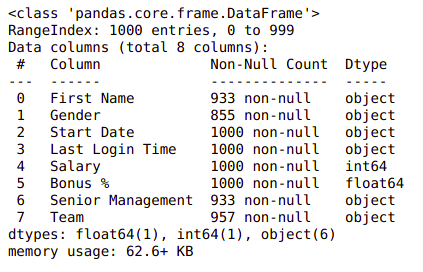Till now we have got an idea about the dataset used. Now Let’s see if our dataset contains any missing value or not.

## Handling Missing Values

You all must be wondering why a dataset will contain any missing value. It can occur when no information is provided for one or more items or for a whole unit. For Example, Suppose different users being surveyed may choose not to share their income, some users may choose not to share the address in this way many datasets went missing. Missing Data is a very big problem in real-life scenarios. Missing Data can also refer to as NA(Not Available) values in pandas. There are several useful functions for detecting, removing, and replacing null values in Pandas DataFrame :

Now let’s check if there are any missing values in our dataset or not.

Example:

## Python3

 `df.isnull().``sum``()`

Output: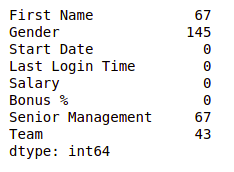We can see that every column has a different amount of missing values. Like Gender as 145 missing values and salary has 0. Now for handling these missing values there can be several cases like dropping the rows containing NaN or replacing NaN with either mean, median, mode, or some other value.

Now, let’s try to fill the missing values of gender with the string “No Gender”.

Example:

## Python3

 `df[``"Gender"``].fillna(``"No Gender"``, inplace ``=` `True``) ``   ` `df.isnull().``sum``()`

Output: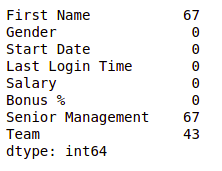We can see that now there is no null value for the gender column. Now, Let’s fill the senior management with the mode value.

Example:

## Python3

 `mode ``=` `df[``'Senior Management'``].mode().values[``0``]``df[``'Senior Management'``]``=` `df[``'Senior Management'``].replace(np.nan, mode)`` ` `df.isnull().``sum``()`

Output: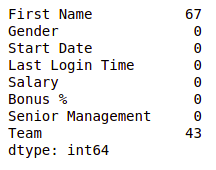Now for the first name and team, we cannot fill the missing values with arbitrary data, so, let’s drop all the rows containing these missing values.

Example:

## Python3

 `df ``=` `df.dropna(axis ``=` `0``, how ``=``'any'``)`` ` `print``(df.isnull().``sum``())``df.shape`

Output: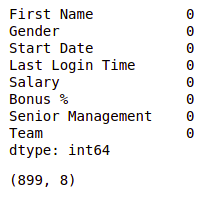We can see that our dataset is now free of all the missing values and after dropping the data the number of also reduced from 1000 to 899.

After removing the missing data let’s visualize our data.

## Data visualization

Data Visualization is the process of analyzing data in the form of graphs or maps, making it a lot easier to understand the trends or patterns in the data. There are various types of visualizations –

• Univariate analysis: This type of data consists of only one variable. The analysis of univariate data is thus the simplest form of analysis since the information deals with only one quantity that changes. It does not deal with causes or relationships and the main purpose of the analysis is to describe the data and find patterns that exist within it.
• Bi-Variate analysis: This type of data involves two different variables. The analysis of this type of data deals with causes and relationships and the analysis is done to find out the relationship among the two variables.
• Multi-Variate analysis: When the data involves three or more variables, it is categorized under multivariate.

Let’s see some commonly used graphs –

Note: We will use Matplotlib and Seaborn library for the data visulalization. If you want to know about these modules refer to the articles –

### Histogram

It can be used for both uni and bivariate analysis.

Example:

## Python3

 `# importing packages``import` `seaborn as sns``import` `matplotlib.pyplot as plt`` ` ` ` `sns.histplot(x``=``'Salary'``, data``=``df, )``plt.show()`

Output: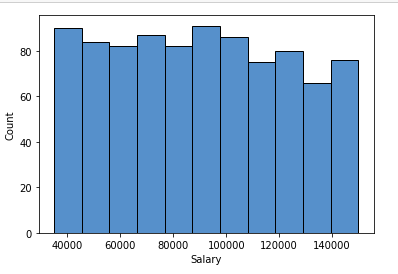### Boxplot

It can also be used for univariate and bivariate analyses.

Example:

## Python3

 `# importing packages``import` `seaborn as sns``import` `matplotlib.pyplot as plt`` ` ` ` `sns.boxplot( x``=``"Salary"``, y``=``'Team'``, data``=``df, )``plt.show()`

Output: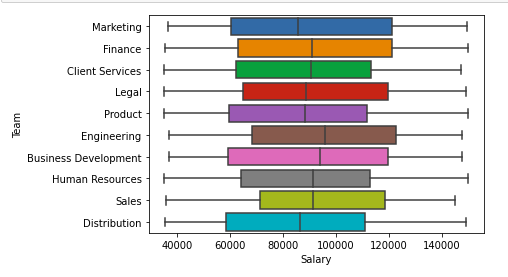### Scatter Plot

It can be used for bivariate analyses.

Example:

## Python3

 `# importing packages``import` `seaborn as sns``import` `matplotlib.pyplot as plt`` ` ` ` `sns.scatterplot( x``=``"Salary"``, y``=``'Team'``, data``=``df,``                ``hue``=``'Gender'``, size``=``'Bonus %'``)`` ` `# Placing Legend outside the Figure``plt.legend(bbox_to_anchor``=``(``1``, ``1``), loc``=``2``)`` ` `plt.show()`

Output: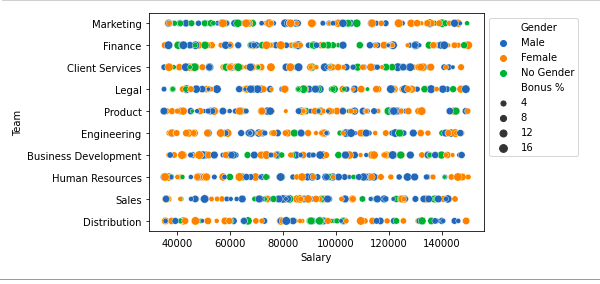For multivariate analysis, we can the pairplot()method of seaborn module. We can also use it for the multiple pairwise bivariate distributions in a dataset.

Example:

## Python3

 `# importing packages``import` `seaborn as sns``import` `matplotlib.pyplot as plt`` ` ` ` `sns.pairplot(df, hue``=``'Gender'``, height``=``2``)`

Output: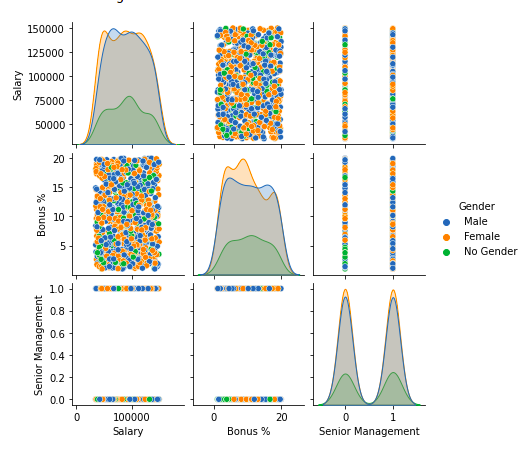## Handling Outliers

An Outlier is a data-item/object that deviates significantly from the rest of the (so-called normal)objects. They can be caused by measurement or execution errors. The analysis for outlier detection is referred to as outlier mining. There are many ways to detect the outliers, and the removal process is the data frame same as removing a data item from the panda’s dataframe.

Let’s consider the iris dataset and let’s plot the boxplot for the SepalWidthCm column.

Example:

## Python3

 `# importing packages``import` `seaborn as sns``import` `matplotlib.pyplot as plt`` ` `# Load the dataset``df ``=` `pd.read_csv(``'Iris.csv'``)`` ` `sns.boxplot(x``=``'SepalWidthCm'``, data``=``df)`

Output: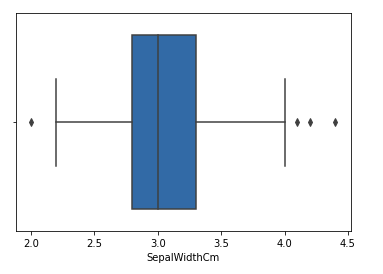In the above graph, the values above 4 and below 2 are acting as outliers.

### Removing Outliers

For removing the outlier, one must follow the same process of removing an entry from the dataset using its exact position in the dataset because in all the above methods of detecting the outliers end result is the list of all those data items that satisfy the outlier definition according to the method used.

Example: We will detect the outliers using IQR and then we will remove them. We will also draw the boxplot to see if the outliers are removed or not.

## Python3

 `# Importing``import` `sklearn``from` `sklearn.datasets ``import` `load_boston``import` `pandas as pd``import` `seaborn as sns`` ` `# Load the dataset``df ``=` `pd.read_csv(``'Iris.csv'``)`` ` `# IQR``Q1 ``=` `np.percentile(df[``'SepalWidthCm'``], ``25``,``                ``interpolation ``=` `'midpoint'``)`` ` `Q3 ``=` `np.percentile(df[``'SepalWidthCm'``], ``75``,``                ``interpolation ``=` `'midpoint'``)``IQR ``=` `Q3 ``-` `Q1`` ` `print``(``"Old Shape: "``, df.shape)`` ` `# Upper bound``upper ``=` `np.where(df[``'SepalWidthCm'``] >``=` `(Q3``+``1.5``*``IQR))`` ` `# Lower bound``lower ``=` `np.where(df[``'SepalWidthCm'``] <``=` `(Q1``-``1.5``*``IQR))`` ` `# Removing the Outliers``df.drop(upper[``0``], inplace ``=` `True``)``df.drop(lower[``0``], inplace ``=` `True``)`` ` `print``(``"New Shape: "``, df.shape)`` ` `sns.boxplot(x``=``'SepalWidthCm'``, data``=``df)`

Output: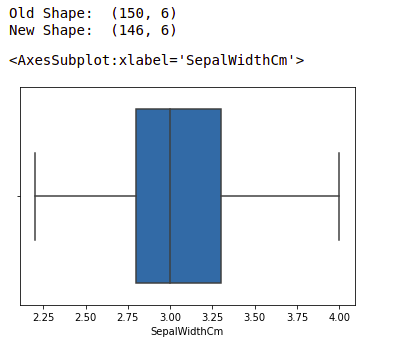Note: for more information, refer Detect and Remove the Outliers using Python

My Personal Notes arrow_drop_up